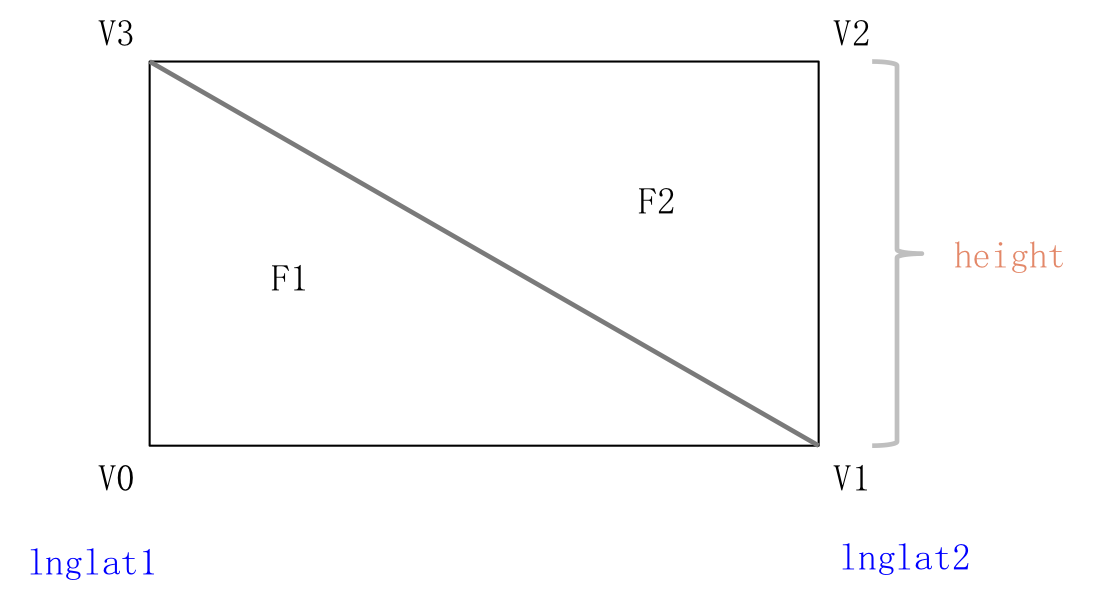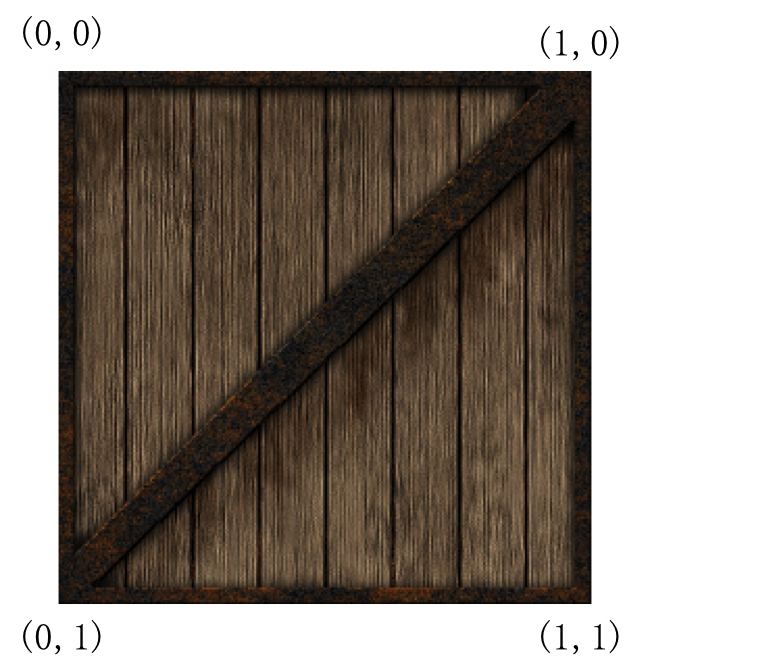# 网格Mesh 最后更新时间: 2021年01月22日

Mesh指的是所有用三角形面组合成的三维物体，JS API 目前提供的 Mesh 类型有：Mesh、MeshAcceptLights、Prism、MeshLine 四种，MeshLine表现为带状线条，放在下一篇介绍

## Mesh 和 MeshAcceptLights

`Mesh``MeshAcceptLights` 为 JS API 提供的比较底层的两个网格类型，默认的图元绘制类型为`gl.TRIANGLES`。以绘制一个垂直于地面的矩形为例，首先我们创建一个Mesh实例：

#### 创建Mesh实例

``````var rectangle = new AMap.Object3D.Mesh()
var geometry = rectangle.geometry;//创建之后获取geometry``````

#### 设置attribute属性

Gometry的属性

`vertices`

`Array<Number>`

`vertexColors`

`Array<Number>`

`faces`

`Array<Integer>`

`vertexUVs`

`Array<Number>`

`textureIndices`

`Array<Integer>`

textureIndices表示每个顶点使用哪个纹理。

`vertexNormals`

`Array<Number>`

`MeshAcceptLights`专用。

``````var lnglat1 = new AMap.LngLat(116.39, 39.9)
var lnglat2 = new AMap.LngLat(116.40, 39.9)
var v0xy = map.lngLatToGeodeticCoord(lnglat1);
var v1xy = map.lngLatToGeodeticCoord(lnglat2);
var z = -1000;//3D地图Z方向朝下，所以负值
geometry.vertices.push(v0xy.x, v0xy.y, 0);//V0
geometry.vertices.push(v1xy.x, v1xy.y, 0);//V1
geometry.vertices.push(v0xy.x, v0xy.y, z);//V3
geometry.vertices.push(v1xy.x, v1xy.y, z);//V2
````````````geometry.faces.push(0, 1, 3)
geometry.faces.push(1, 2, 3)``````

``````geometry.vertexColors.push(1, 0, 0, 1); //V0
geometry.vertexColors.push(0, 1, 0, 1); //V1
geometry.vertexColors.push(0, 0, 1, 1); //V2
geometry.vertexColors.push(0, 1, 1, 1); //V3

rectangle.transparent = false;``````

``rectangle.textures.push("https://a.amap.com/jsapi_demos/static/tourist/crate.gif");``

x轴朝右，y轴朝下：

``````
geometry.vertexUVs.push(0, 1); //V0
geometry.vertexUVs.push(1, 1); //V1
geometry.vertexUVs.push(1, 0); //V2
geometry.vertexUVs.push(0, 0); //V3

```````vertexNormals``MeshAcceptLights`专有的属性，用作记录顶点法向量，来实现光照，这里不细说明。

#### 添加到 Object3DLayer

``````rectangle.backOrFront = 'both';//'back'、'front'、'both'

## 棱柱 Prism

``````var bounds = [
new AMap.LngLat(116.5,39.25),
new AMap.LngLat(116.75,39.5),
new AMap.LngLat(116.5,39.75),
new AMap.LngLat(116.25,39.5)
]
var height = 500000;
var color = [0,0,1,0.6];
var prism2 = new AMap.Object3D.Prism({
path:island,
height:height,
color:color
});``````